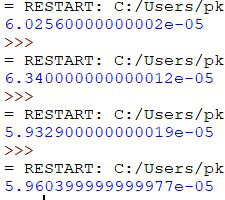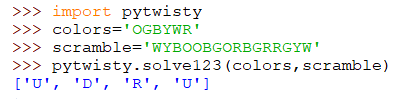# pytwisty: Rubik’s Cube Solver – Python Project

• Last Updated : 18 Aug, 2021

pytwisty is an extremely fast and efficient Python 3 implementation of a solver for a number of twisty puzzles including the 1x2x2, 1x2x3, and 2x2x2 Rubik’s cube puzzles.

## Introduction

Modern speedcubers solve the Rubik’s cube using memorized sequences of moves, called algorithms, which they deploy to solve the cube section by section. Each algorithm corresponds to a different arrangement of colored stickers on the cube. When a speedcuber spots an arrangement he recognizes, he performs the corresponding algorithm, bringing the cube one step closer to solving.

Attention geek! Strengthen your foundations with the Python Programming Foundation Course and learn the basics.

To begin with, your interview preparations Enhance your Data Structures concepts with the Python DS Course. And to begin with your Machine Learning Journey, join the Machine Learning - Basic Level Course

However, conventional computerized solvers simply look for an optimal solution from subsets of all possible positions of the pieces on the cube using complex search techniques. The fastest one of these is Kociemba’s algorithm which identifies a subset of 20 billion positions. This algorithm was in fact also used to calculate the solution in the robot that holds the current Guinness World Record for the fastest solve by a robot. For experimentation, we have compared the 2x2x2 solver developed by Herbert Kociemba himself on my machine with two other implementations of Korf’s Algorithm and the 2-way BFS method of solving the cube, both of which are also among the fastest solving methods out there. Upon measuring their average solving times, Kociemba’s solver indeed proved to be the fastest among them.

By contrast, we will develop a solver that uses the “human approach” to solve the puzzle. Specifically, it runs a combination of a slightly altered version of the layer-by-layer (LBL) and the CFOP method. When we ran a 2x2x2 solver on the same set of random scrambles, it solve time on average was about 350 times faster than the solves times of the Herbert Kociemba solver mentioned above. We used the timeit module to measure the solve times of the solver and the mean solves time in seconds turned out to be of the order 10^-5 sec whereas the time taken by the Kociemba’s solver in seconds was of the order 10^-2 sec on my machine on CPython.## Implementation

The main reason behind the extremely high speed and efficiency of the solver is that it is based on a technique developed which I like to call the “Minimal Thinking” technique. This, in simplest terms, is a technique that minimizes the thought process behind solving any problem by recognizing the type of problem and using a predetermined result to jump straight to the solution. At each step of solving the puzzle, the solver recognizes the current arrangement and orientation of the individual pieces of the puzzle, and rearranges and reorients the pieces into the final state that the corresponding algorithm would have resulted in, had it been manually executed. This is just like how speedcubers, at each level of solving the Rubik’s cube, look at the arrangement of the cube and execute the corresponding algorithm, all without thinking.

Sample Usage:An example of using the solver to solve a random scramble of the 1x2x3 Rubik’s Cube. Here, “OGBYWR” is the color scheme of the user’s Rubik’s Cube and “WYBOOBGORBGRRGYW” is the given scramble. Instructions on how to correctly input the color alignment of one’s cube and the scramble can be found in the PyPI or the GitHub Repository ReadMe File. The 1x2x3 solver outputs a series of steps: [‘U’, ‘D’, ‘R’, ‘U’]. Each step would be of the form U, D, R, L, or M each of which represent a 180-degree turn of the upper layer, bottom layer, right face, left face, and middle layer respectively. When the user will execute these steps on his cube, he will get a solved cube.

## Applications

This Rubik’s cube solver is just one of the several applications that the Minimal Thinking technique can potentially have in modern Artificial Intelligence. It can be used to ease the problem-solving part of every program by training it to use results that have already been established both by human or artificial intelligence and directly jumping to the solution without having to solve the entire problem on its own. Although the “human method” of solving the Rubik’s cube comes with the disadvantage of not always providing the optimal solution, the 350x boost in program efficiency effectively outweighs any such potential disadvantages. For modern robots that execute each move on a physical Rubik’s cube in as little as 10 milliseconds, executing a handful of extra moves is an almost negligible task. In most real-life problems, an optimal solution may not always prove to be the best solution, especially when it is generated at the cost of an extremely slow and excessively memory-consuming program. Regardless of how modern AI has advanced to a point where robots tend to have the potential to “think” on their own, even a small human interaction in a completely autonomous program can make a huge difference in its efficiency, as made evident in this example of a Rubik’s cube solver.